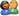登  录风  格绿草青青青青苹果蓝色经典佳人有约蓝色梦想蓝天白云蓝色狂想蓝色魅力魅力星空
 »  游客当前位置：  论坛首页 »  自由讨论区 »  Android, Python及开发编程讨论区 »总帖数 1 每页帖数 10 1/1页 1
0查看: 6947 | 回复: 0 主题： [转帖]JAVA经典算法几例（1）liulin_2 注册用户等级：少校 经验：1143 发帖：85 精华：5 注册：2012-12-10 状态：离线加好友发消息

 【程序 1】 题目：古典问题：有一对兔子，从出生后第3个月起每个月都生一对兔子，小兔子长到第四个月后每个月又生一对兔子，假如兔子都不死，问每个月的兔子总数为多少？ 1. 程序分析： 兔子的规律为数列1,1,2,3,5,8,13,21.... public class exp2{      public static void main(String args[]){            int i=0;            for(i=1;i<=20;i++)            System.out.println(f(i));      }      public static int f(int x)      {            if(x==1 || x==2)            return 1;            else            return f(x-1)+f(x-2);      }}或 public class exp2{      public static void main(String args[]){            int i=0;            math mymath = new math();            for(i=1;i<=20;i++)            System.out.println(mymath.f(i));      }}class math{public int f(int x){      if(x==1 || x==2)      return 1;      else      return f(x-1)+f(x-2);}}【程序 2】 题目：判断101-200之间有多少个素数，并输出所有素数。 1. 程序分析：判断素数的方法：用一个数分别去除2到sqrt(这个数)，如果能被整除， 则表明此数不是素数，反之是素数。  public class exp2{      public static void main(String args[]){            int i=0;            math mymath = new math();            for(i=2;i<=200;i++)            if(mymath.iszhishu(i)==true)            System.out.println(i);      }}class math{public boolean iszhishu(int x){      for(int i=2;i<=x/2;i++)      if (x % i==0 )      return false;      return true;}}【程序 3】 题目：打印出所有的 "水仙花数 "，所谓 "水仙花数 "是指一个三位数，其各位数字立方和等于该数本身。例如：153是一个 "水仙花数 "，因为153=1的三次方＋5的三次方＋3的三次方。 1. 程序分析：利用for循环控制100-999个数，每个数分解出个位，十位，百位。  public class exp2{      public static void main(String args[]){            int i=0;            math mymath = new math();            for(i=100;i<=999;i++)            if(mymath.shuixianhua(i)==true)            System.out.println(i);      }}class math{public int f(int x){      if(x==1 || x==2)      return 1;      else      return f(x-1)+f(x-2);}public boolean iszhishu(int x){      for(int i=2;i<=x/2;i++)      if (x % 2==0 )      return false;      return true;}public boolean shuixianhua(int x){      int i=0,j=0,k=0;      i=x / 100;      j=(x % 100) /10;      k=x % 10;      if(x==i*i*i+j*j*j+k*k*k)      return true;      else      return false;}}【程序 4】 题目：将一个正整数分解质因数。例如：输入90,打印出90=2*3*3*5。 程序分析：对 n进行分解质因数，应先找到一个最小的质数k，然后按下述步骤完成： (1) 如果这个质数恰等于n，则说明分解质因数的过程已经结束，打印出即可。 (2) 如果n <> k，但n能被k整除，则应打印出k的值，并用n除以k的商,作为新的正整数你,重复执行第一步。 (3) 如果n不能被k整除，则用k+1作为k的值,重复执行第一步。  public class exp2{public exp2(){}      public void fengjie(int n){            for(int i=2;i<=n/2;i++){                  if(n%i==0){                        System.out.print(i+"*");                        fengjie(n/i);                  }            }            System.out.print(n);            System.exit(0);/// 不能少这句，否则结果会出错      }      public static void main(String[] args){            String str="";            exp2 c=new exp2();            str=javax.swing.JOptionPane.showInputDialog(" 请输入N的值（输入exit退出）：");            int N;            N=0;            try{                  N=Integer.parseInt(str);            }catch(NumberFormatException e){            e.printStackTrace();      }      System.out.print(N+" 分解质因数："+N+"=");      c.fengjie(N);}}【程序 5】 题目：利用条件运算符的嵌套来完成此题：学习成绩> =90分的同学用A表示，60-89分之间的用B表示，60分以下的用C表示。 1. 程序分析：(a> b)?a:b这是条件运算符的基本例子。  import javax.swing.*;public class ex5 {      public static void main(String[] args){            String str="";            str=JOptionPane.showInputDialog(" 请输入N的值（输入exit退出）：");            int N;            N=0;            try{                  N=Integer.parseInt(str);            }            catch(NumberFormatException e){                  e.printStackTrace();            }            str=(N>90?"A":(N>60?"B":"C"));            System.out.println(str);      }}

 本版精华 热门帖子操作    引用/回复总帖数 1 每页帖数 10 1/1页 1请输入验证码：您需要登录后才可以回帖 登录 | 注册技术讨论